# Keeping Distance

For which values of n can n points be placed on a sphere so that all of them are equidistant from each other?

# Some Two

Given 52 integers, prove that it’s always possible to find some two of them whose sum or difference is evenly divisible by 100.

# Black and White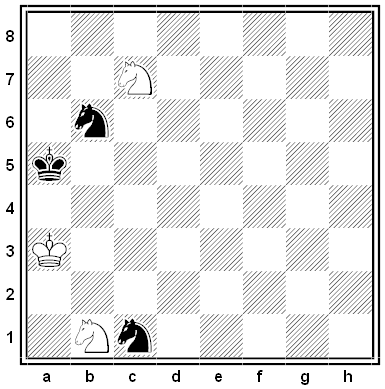From Louis Friedländer’s 1919 collection Chess Poetry. White to mate in two moves.

# Black and White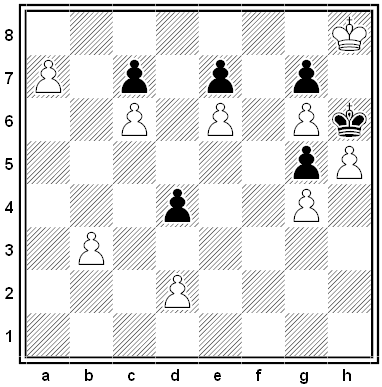A remarkable thematic chess puzzle by Bodo Van Dehn, 1951. White to move and win.

The solution is 10 moves long, but all Black’s moves are forced. (That’s a very valuable hint.)

# A Flea’s Journey

A flea sits on one vertex of a regular tetrahedron. He hops continually from one vertex to another, resting for a minute between hops and choosing vertices without bias. Prove that, counting the first hop, we’d expect him to return to his starting point after four hops.

# The Magic Total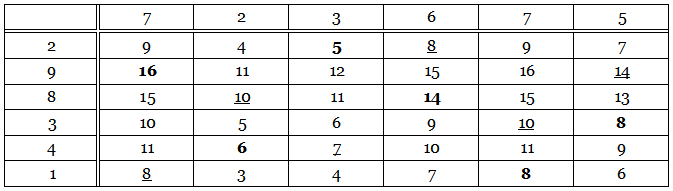Each of the 36 numbers in this table is the sum of the numbers at the head of its column and at the left of its row. For example, 3 = 2 + 1 and 13 = 5 + 8. The six bold numbers have been chosen so that each of them falls in a different row and a different column. The underlined numbers were chosen in the same way. But each of these two sextets produces the same total: 16 + 6 + 5 + 14 + 8 + 8 = 8 + 10 + 7 + 8 + 10 + 14 = 57. In fact, any six numbers chosen in this way will produce the total 57. Why is this?

# Home Again

On a regular 8 × 8 chessboard, a wandering knight can visit each square once and then return to his starting square. Show that he can’t do this on an m × n board if m and n are both odd.

# Black and White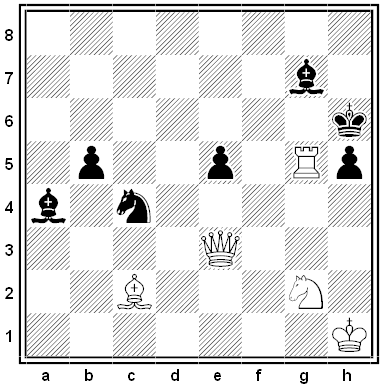By Francis Healey. White to mate in two moves.

# Black and White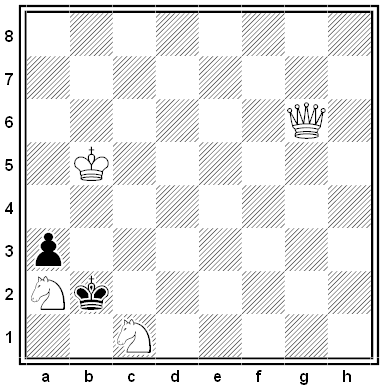This problem, by Charles Pelle, won third prize among the Union des Problémistes de France in 1946. How can White mate in two moves?

# Simple Enough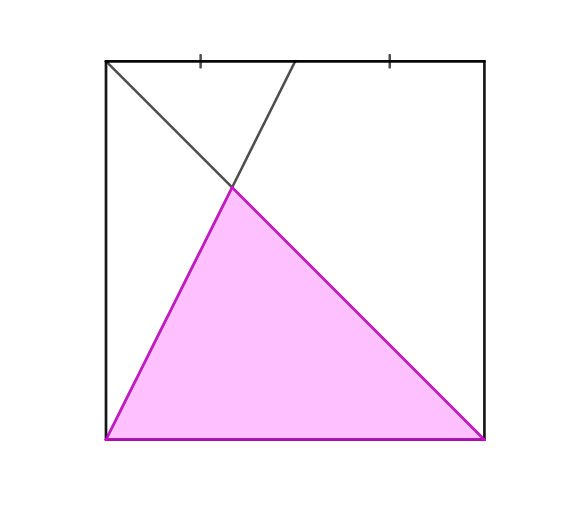I think this appeared originally in Ed Southall’s Twitter feed. What fraction of the square is shaded?